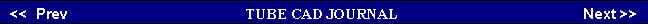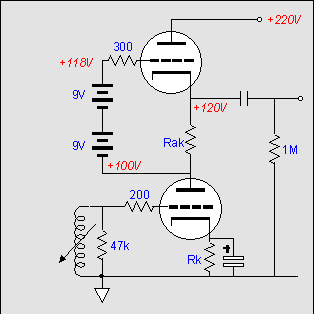Here is a trivia question: How large a value of cathode resistor is needed to completely turn off the 12AX7? A trick question doubtless, for no cathode resistor can be large enough to turn off the tube, as the only way a negative bias voltage can develop across the resistor is when the tube conducts -- which means it cannot be turned off. While we are on the topic of tricks, one strategy for increasing the effective load impedance of the active load without having to resort to an extra coupling capacitor is to run the triodes dissimilarly. Normally, Rak would equal Rk, but they can differ in value. Using different values means that the triodes will see different cathode-to-plate voltages, while seeing the same current. For example, equal resistor values would yield an equal voltage across each triode. Increasing Rak's value will increase the voltage across the top triode, but diminish the voltage across the bottom triode. Given a 200-volts B+ power supply, a good ratio might be 130-volts for the top tube and 70-volts for the bottom tube.      Thus, we have increased the value of Rak, and the effective impedance of the active load at the same time, without having resorted to adding an extra capacitor. However, if we desire the highest gain, the most linearity, and the lowest amount of power supply noise at the output, then a coupling capacitor must be added, as that is simply the price we pay for using a much larger value for the resistor Rak.

Actively loaded (constant current source) grounded-cathode amplifier
 Actively Loaded Grounded-Cathode   Let's move slowly through some of the possible candidates for the first gain stage. The simplest variation on the grounded-cathode amplifier we have already covered, entailing using more triodes in parallel. Moving up in complexity, the actively-loaded grounded-cathode amplifier finds its plate resistor replaced by another triode that functions as an active load. Depending on the size of its cathode resistor, the effective impedance this load presents to the bottom triode can easily be much larger than the plate resistor it replaces. The formula is a simple one:     Z = rp + (µ + 1)Rk,where, µ equals the triode's amplification factor (mu). For example, a 12AX7 with 20k cathode resistor would represent an impedance of     2,082,000 = 62k + (100 +1)20,000.Just 1-mA against a resistor with this value would develop a voltage of 2,080-volts! Yet, both triodes could easily operate with a B+ voltage of only 300-volts. Unfortunately, using a 20k cathode resistor with a 12AX7 will require using an extra coupling capacitor, as a 20k resistor would bias the tube down to near cutoff.